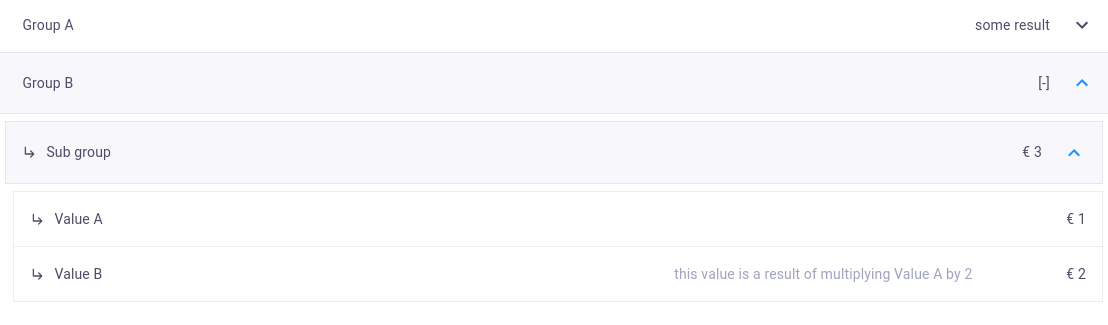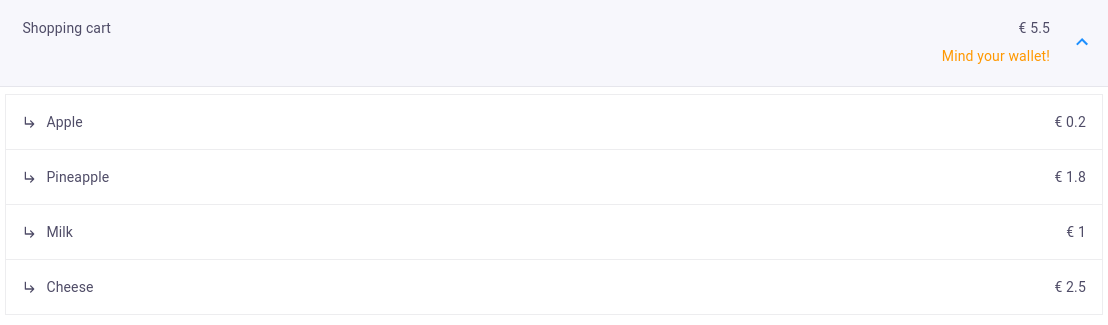# Show data & tables

The `DataView` is a powerful feature, in which, for example, the results of one or more calculations can be shown immediately (e.g. the volume of an object). The entries in a `DataView` can be divided into three levels.Data view

## Implementation​

The following steps have to be performed to visualize the desired results:

1. Create the data group(s) consisting of `DataItems` for visualization.
2. Pass the group(s) to a decorated view method that returns a `DataResult`.
``from viktor.views import DataGroup, DataItem, DataResult, DataViewclass Controller(ViktorController):    ...    @DataView("OUTPUT", duration_guess=1)    def visualize_data(self, params, **kwargs):        data = DataGroup(            DataItem('Data item 1', 123)        )        return DataResult(data)``

## Multilayered DataGroup​

Below an example is given of a multilayered output group (maximum of three layers deep):

``@DataView("OUTPUT", duration_guess=1)def visualize_data(self, params, **kwargs):    value_a = 1    value_b = 2 * value_a    value_total = value_a + value_b    data = DataGroup(        group_a=DataItem('Group A', 'some result', subgroup=DataGroup(            sub_group=DataItem('Result', 1, suffix='N')        )),        group_b=DataItem('Group B', '', subgroup=DataGroup(            sub_group=DataItem('Sub group', value_total, prefix='€', subgroup=DataGroup(                value_a=DataItem('Value A', value_a, prefix='€'),                value_b=DataItem('Value B', value_b, prefix='€', explanation_label='this value is a result of multiplying Value A by 2')            ))        ))    )    return DataResult(data)``

The `explanation_label` can be used to inform the user. The output result will look like this:Example DataView

## Positional or key-worded DataItem?​

In previous examples, we saw a DataGroup created with and without the use of keyword arguments. Using keywords is necessary to link the corresponding `DataItem` to the `Summary`. A detailed guide of the `Summary` can be found here.

## Dynamic group length​

The structure of `DataGroup`s allows for dynamic creation/addition of data to the overall result, as long as the maximum number of items is not exceeded. An example of dynamically creating data items is shown below:

``shopping_cart = {    'Apple': 0.20,    'Pineapple': 1.80,    'Milk': 1}# loop through productstotal_cost = 0data_items = []for item, price in shopping_cart.items():    data_items.append(DataItem(item, price, prefix='€'))    total_cost += price# construct data groupdata_group = DataGroup(    DataItem('Shopping cart', total_cost, prefix='€', subgroup=DataGroup(*data_items)))``

## Setting warning/error statuses​

Sometimes it is desired to warn a user when a result exceeds a certain values. For these cases, the `status` and `status_message` arguments of a `DataItem` can be considered. Suppose we want to warn the user if our shopping cart of the example above exceeds 5 euros, we can add the following:

``shopping_cart.update({    'Cheese': 2.50})# loop through products...# construct data groupif total_cost <= 5:    status = DataStatus.SUCCESS    status_msg = ''else:    status = DataStatus.WARNING    status_msg = 'Mind your wallet!'data_group = DataGroup(    DataItem('Shopping cart', total_cost, prefix='€', status=status, status_message=status_msg, subgroup=DataGroup(*data_items)))``Example status message

## Testing​

New in v13.3.0

`mock_View` decorator for easier testing of view methods

Methods decorated with `@DataView` need to be mocked within the context of (automated) testing:

``import unittestfrom viktor.testing import mock_Viewfrom app.my_entity_type.controller import MyEntityTypeControllerclass TestMyEntityTypeController(unittest.TestCase):    @mock_View(MyEntityTypeController)    def test_data_view(self):        params = ...        result = MyEntityTypeController().data_view(params=params)        self.assertEqual(result.data, ...)``